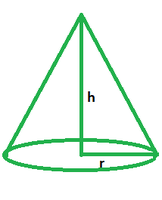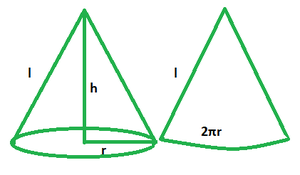# Surface Area of a Cone Formula

A geometric object with a smooth transition from a flat base to the apex or vertex is called a cone. It consists of segments, split lines, or lines that link a commonality, the pinnacle, to most of the spots on a base that are not in the same plane as the apex.### Surface Area of Cone

The surface area of a cone is the area filled by the outer edge of a cone. It is usually expressed in terms of square units. The form of a cone is created by stacking numerous triangles and spinning them around an axis. It has a total surface area as well as a curved surface area because it has a flat base. A cone can be classified as either a right circular or an oblique cone. In a right circular cone, the vertex is normally vertically above the centre of the base, but in an oblique cone, the vertex is not vertically above the centre of the base.

Formula

TSA = πr(r + l) or πr(r + √(r2 + h2))

where h denotes the height of the cone and r is the radius and l is the slant height.

Derivation

Assume a cone of height, radius and slant height h, r and l respectively.

Cut it from the center so it is converted into a 2D shape.TSA of cone = CSA + base area

= πr2 + πrl

= πr (r + l)

Hence proved.

### Sample Problems

Problem 1. Find the TSA of a cone if its radius and slant height are 4 cm and 5 cm.

Solution:

Given: r = 4 cm

l = 5 cm

TSA = πr (r + l)

= 3.14 x 4(4 + 5)

= 113.04 cm2

Problem 2. Find the TSA of a cone if its slant height is 8 cm and radius is 2 cm.

Solution:

Given: r = 2 cm

l = 8 cm

TSA = πr (r + l)

= 3.14 x 2(2 + 8)

= 62.8 cm2

Problem 3. Find the slant height of a cone if its TSA is 616 cm2 and radius 7 cm.

Solution:

Given: TSA = 616 cm2

r = 7 cm

Let the slant height be l cm.

Since TSA of a cone = πr (r + l)

⇒ 616 = 22x7x (7 + l)/7

⇒ 7 + l = 616/22

l = 28 – 7

l = 21 cm

Problem 4. Find the TSA of a cone with a radius of 14 cm and slant height of 8 cm.

Solution:

Given: r = 14 cm

l = 8 cm

TSA = πr (r + l)

= 3.14 x 14(14 + 8)

= 967.12 cm2

Problem 5. Find the TSA of a cone with a radius of 5 cm and slant height of 8 cm.

Solution:

Given: r = 5 cm

l = 8 cm

TSA = πr (r + l)

= 3.14 x 5(5 + 8)

= 204.10 cm2

Whether you're preparing for your first job interview or aiming to upskill in this ever-evolving tech landscape, GeeksforGeeks Courses are your key to success. We provide top-quality content at affordable prices, all geared towards accelerating your growth in a time-bound manner. Join the millions we've already empowered, and we're here to do the same for you. Don't miss out - check it out now!

Related Tutorials